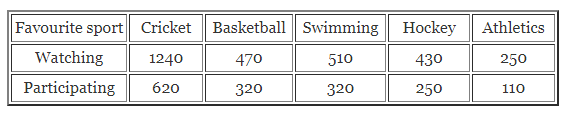# ML Aggarwal Class 7 Solutions for ICSE Maths Chapter 17 Data Handling Ex 17.1

## ML Aggarwal Class 7 Solutions for ICSE Maths Chapter 17 Data Handling Ex 17.1

Question 1.
The number of rooms in 25 houses of a locality is as given below:
4, 3, 2, 6, 4, 3, 2, 1, 5, 3, 2, 3, 4, 3, 5, 1, 6, 1, 3, 4, 2, 3, 4, 3, 5
(i) Arrange the above data in ascending order.
(ii) Find the range of the data.
(iii) Construct a frequency distribution table for the above data.
(iv) Find the number of houses which have 4 or more than 4 rooms.
(v) Drawbar graph to represent the above data.
Solution: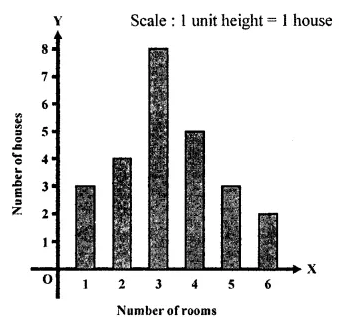Question 2.
The given bar graph shows the number of books sold by a bookstore for five consecutive years. Read the bar graph and answer the following questions:
(i) About how many books were sold in 2008, 2009 and 2011 years?
(ii) In which years were about 475 books and 225 books sold?Solution: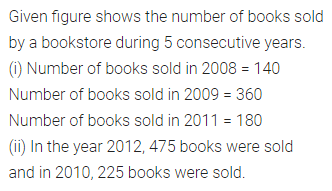Question 3.
Two hundred students of 6th and 7th class were asked to name their favorite colour so as to decide upon what should be the colour of their school building. The results are shown in the following table: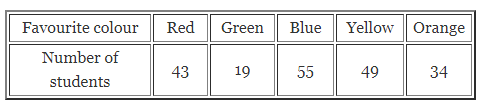Represent the given data on a bar graph.
Answer the following questions with the help of the bar graph:
(i) Which is the most preferred colour?
(ii) Which is the least preferred colour?
(iii) How many colours are there in all? What are they?
Solution: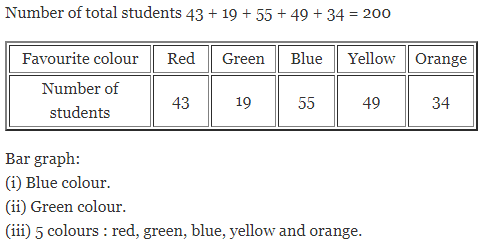Question 4.
Sale of English and Hindi books in four consecutive years is given below:Draw a double bar graph to represent the above data and answer the following questions:
(i) In which year was the difference in the sale of two language books least?
(ii) Can you say that the demand for English books rose faster? Justify your answer.
Solution: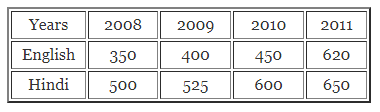Question 5.
Consider the following data collected from a survey of a colony:Draw a double bar graph choosing an appropriate scale.
Answer the following questions using the bar graph:
(i) Which sports is most popular?
(ii) Which is more preferred, watching or participating in sports?
Solution: